## Welcome to the OpenCyto workshop!

### Data

The `tbdata` GatingSet is part of a data set of intracellular cytokine staining (ICS) samples from TB-infected and non-infected subjects, stimulated with TB-specific and non-specific peptides. The samples were assayed for Ag-specific response to stimulation. The data were analyzed in Lin et al. (2015), using results from manual gating.

### The data structures and objects underlying OpenCyto and the BioConductor Flow Cytometry Infrastructure

1. `flowFrame` is an R object that corresponds to a single FCS file.
2. `flowSet` is a collection of `flowFrame`s, all with the same markers and channels.
3. `GatingHierarchy` is a `flowFrame` with its associated data transformation, compensation, and gating information defining cell populations.
4. `GatingSet` is a collection of `GatingHierarchy` objects, or equivalently a `flowSet`, with all transformation, compensation, and gating information.

#### Gating information stored in a GatingSet

The GatingSet associates each sample with a hierarchy of gates that define cell popualtions. The gates can be one, two, three or high-dimensional. Any gates supported by the `flowCore` infrastructure can be attached to a a hierarchy of gates in a `GatingSet`.

The GatingSet lets us visualize FCM data analysis at the individual event-level (single-cells). Data are stored on disk using HDF5 and are read-in as needed. This has a very efficient memory footprint and allows us to analyze large data sets on modest hardware.

It provides a conveninent and unified way to interact with FCM data for performing hierarchical or non-hierarchical automated gating by managing cell population definitions and their relationships.

If your aren’t yet using this infrastructure, you should be.

#### OpenCyto

There are at least 50 different algorithms in the literature for performing automated gating of FCM data. 75% of those are available for BioConductor.

There is no “one best algorithm” for FCM gating. Different algorithms have different strengths (Aghaeepour2013a).

OpenCyto provides a mechansim to use multiple algorithms to identify different cell populations. Complex high-dimensional methods are rarely necessary, and the focus is on simple methods that work robustly rather than fancy approaches that work on one data set.

#### Gating Templates

The general idea is that for a given FCM assay utilizing a set staining panel generated by one lab, a general strategy to identify cell populations of interest should be applicable to any set of data produced by the lab.

OpenCyto formalizes this in the form of a `gatingTemplate`. This template defines cell populations in terms of their phenotypic markers, parent populations, and gating methods / algorithms used to define them. The template is in the form of a `csv` file that is easier to create than an R script. openCyto will take the template and a data set and produce data-driven gates for each cell population and sample.

#### Let’s get started!

Our goal here will be to gate the `tbdata` data set. We have multiple samples per subject. One sample is unstimulated, the other is stimulated with ESAT-6 peptide. We’ll focus on identifying antigen-specific CD4+ T cells.

To this end, we need to compare the stimulated and non-stimulated samples within each subject.

#### Cleaning up the data.

The data were imported from a manual gating analysis. We’ll begin by deleting the manual gates, as we’re not interested in them for this demo. We’ll also rename some phenotypic data columns so that we can use faceting more easily.

The general gating scheme we’ll apply here is `root/Singlets/CD14-/nonDebris/Lymphocytes/Live/CD3/CD4/Functional markers`.

``````#Delete existing gates
Rm("Singlets",tbdata)``````

Next, we’ll use the new API `add_pop` in openCyto to build our new automated gating strategy.

We’ll grab a subset of the data for testing.

``tbdata_subset = subset(tbdata,`PID`%in%unique(pData(tbdata)\$`PID`)[1:2])``

We’ll build up our gating template incrementally.

``````template = add_pop(
tbdata_subset, alias = "Singlets", pop = "Singlets", parent = "root", dims = "FSC-A,FSC-H",gating_method = "singletGate",gating_args =
"wider_gate=TRUE,subsample_pct=0.1"
)``````

Plotting it, we see it looks reasonable.

``````ggcyto(tbdata_subset,mapping = aes(x = `FSC-A`,y = `FSC-H`)) + geom_hex(bins =
50) + geom_gate("Singlets") + xlim(c(0,2e5))``````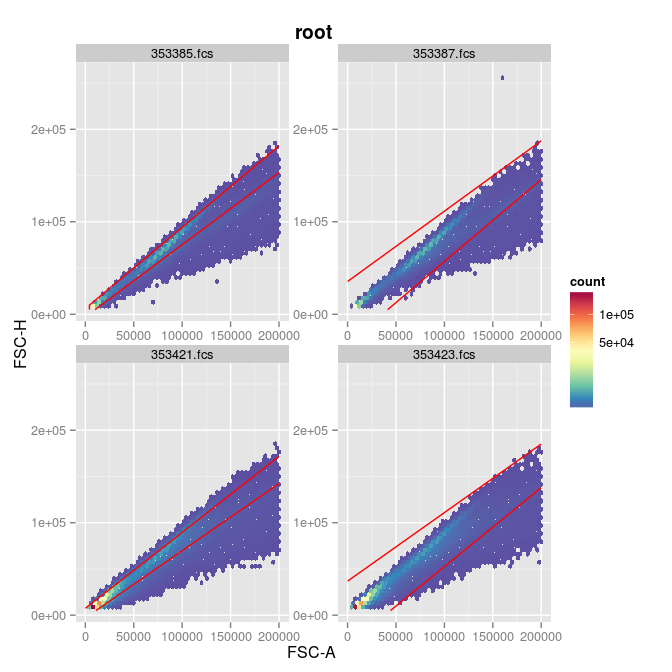#### CD14- Cells

Next we add CD14- cells. Lets’ see what this dimension looks like and decide on a good gating method.

``````ggcyto(tbdata_subset,mapping = aes(x = "CD14"),subset = "Singlets") + geom_histogram(binwidth =
100) + xlim(c(-100,4096)) + facet_wrap( ~ PID,scales = "free")``````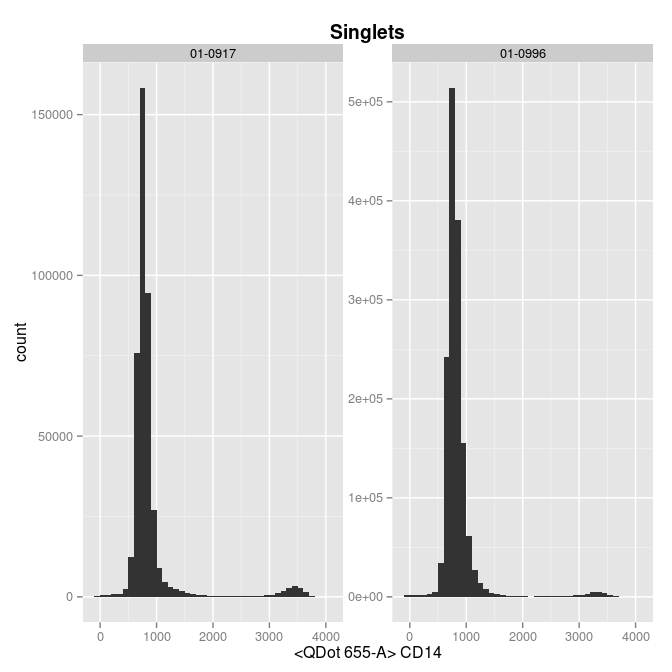Mindensity should be able to handle this easily.

#### mindensity method on CD14-

``````template = rbind(
tbdata_subset,alias = "CD14n", "CD14-", parent = "Singlets", dims = "CD14", gating_method =
"mindensity",groupBy = "PID",collapseDataForGating = TRUE
)
)``````
``````ggcyto(tbdata_subset,mapping = aes(x = "CD14"),subset = "Singlets") + geom_histogram(binwidth =
100) + xlim(c(-100,4096)) + facet_wrap( ~ PID,scales = "free") + geom_gate("CD14n")``````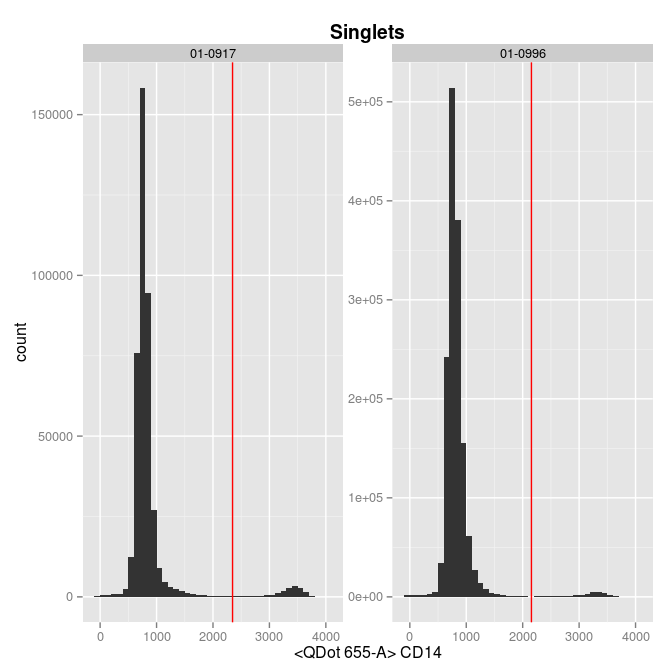### Next we gate out debris

``````template = template[1:2,]
template = rbind(
tbdata_subset,alias = "nonDebris",pop = "nonDebris+",parent = "CD14n",dims = "FSC-A",gating_method =
"boundary",collapseDataForGating = FALSE,gating_args = "min=40000,max=2.5e5"
)
)
ggcyto(tbdata_subset,mapping = aes(x = "FSC-A",y = "SSC-A"),subset = "CD14n") + geom_hex(bins = 100) +
geom_gate()``````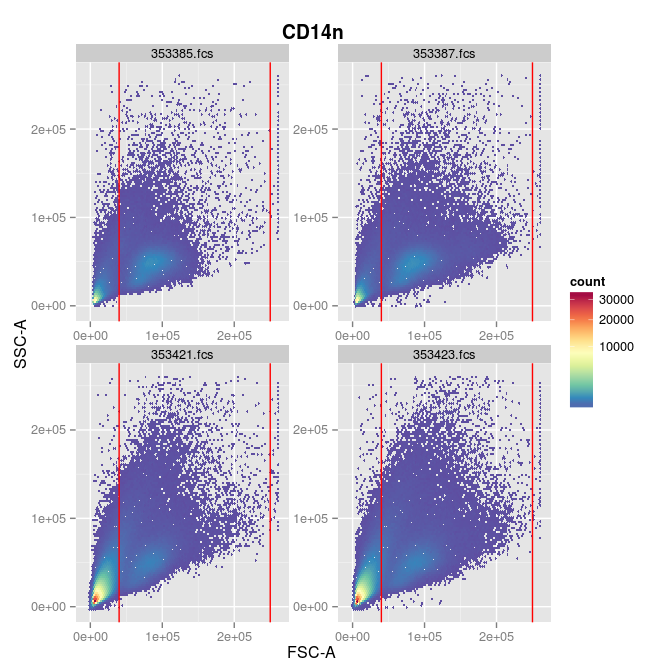### And then lymphocytes

``````  template = rbind(
tbdata_subset,alias = "Lymphocytes",pop = "Lymphocytes+",parent = "nonDebris",dims = "FSC-A,SSC-A",gating_method =
"flowClust.2d",preprocessing_method = "prior_flowClust",preprocessing_args="K=3",collapseDataForGating = FALSE,gating_args =
"quantile=0.95,target=c(80000,50000),K=3"
)
)
ggcyto(tbdata_subset,mapping = aes(x = "FSC-A",y = "SSC-A"),subset = "nonDebris") +
geom_hex(bins = 50) + geom_gate("Lymphocytes")``````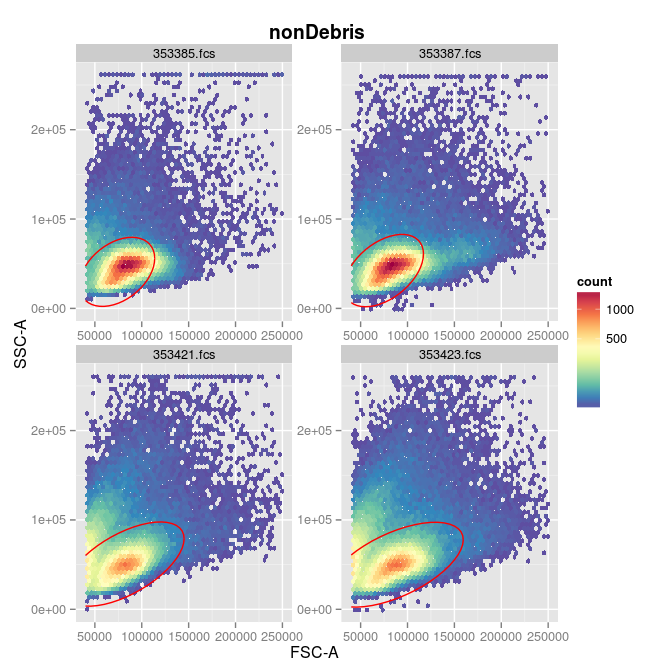### Next we gate live cells.

``````  template = rbind(
tbdata_subset,alias = "Live",pop = "Live+",parent = "Lymphocytes",dims = "<Am Cyan-A>",gating_method = "boundary",gating_args = "min=0,max=2000",collapseDataForGating = FALSE
)
)

ggcyto(tbdata_subset,mapping = aes(x = "AViD",y="SSC-A"),subset = "Lymphocytes") +
geom_hex(bins = 100) + ggcyto_par_set(limits = "instrument") +
geom_gate("Live")``````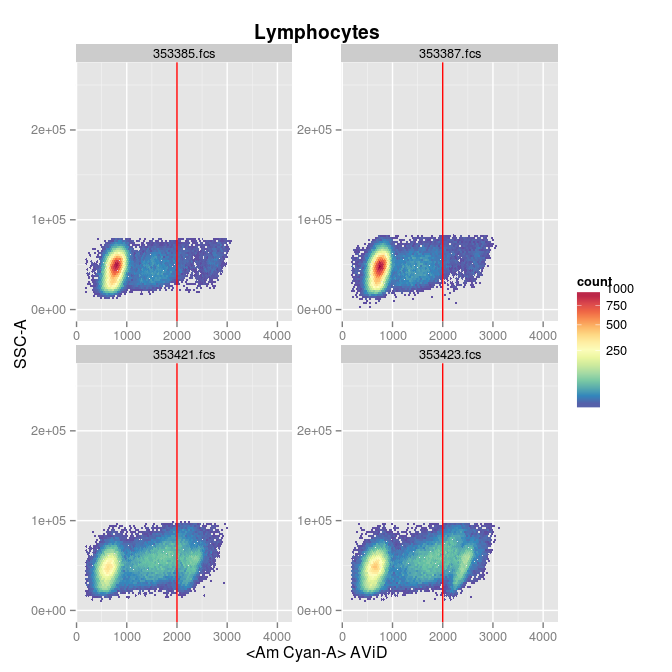### Then CD3+ cells

``````    template = rbind(
tbdata_subset,alias = "CD3",pop = "CD3+",parent = "Live",dims = "CD3",gating_method = "mindensity"
)
)
ggcyto(tbdata_subset,mapping = aes(x = "CD3",y = "FSC-A"),subset = "Live") +
geom_hex(bins=100) + ggcyto_par_set(limits = "instrument") + geom_gate("CD3")``````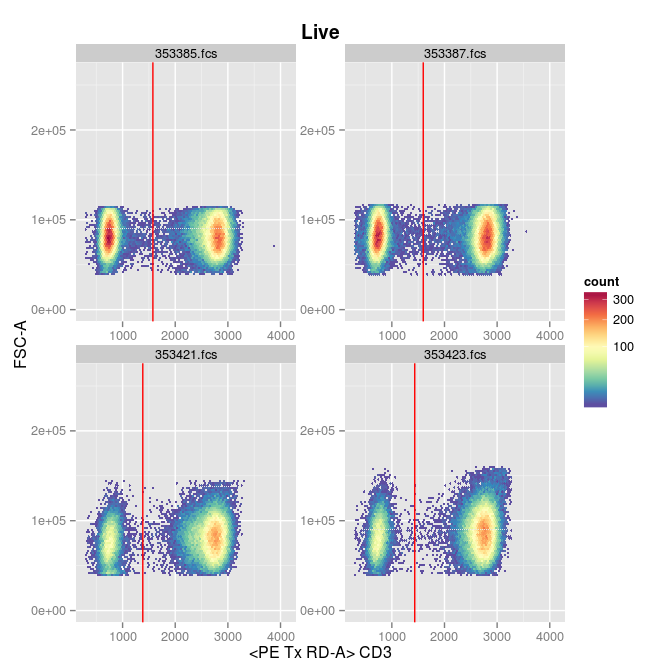### And finally CD4+/CD8- cells

``````      template = rbind(
tbdata_subset,alias = "*",pop = "CD4+/-CD8+/-",dims = "CD4,CD8",gating_method =
"mindensity2",gating_args="gate_range=c(500,4000)",parent = "CD3")
)
ggcyto(tbdata_subset,mapping = aes(x = "CD4",y = "CD8"),subset = "CD3") +
geom_hex(bins=100) + ggcyto_par_set(limits = "data") + geom_gate()+xlim(0,3000)+ylim(0,4000)``````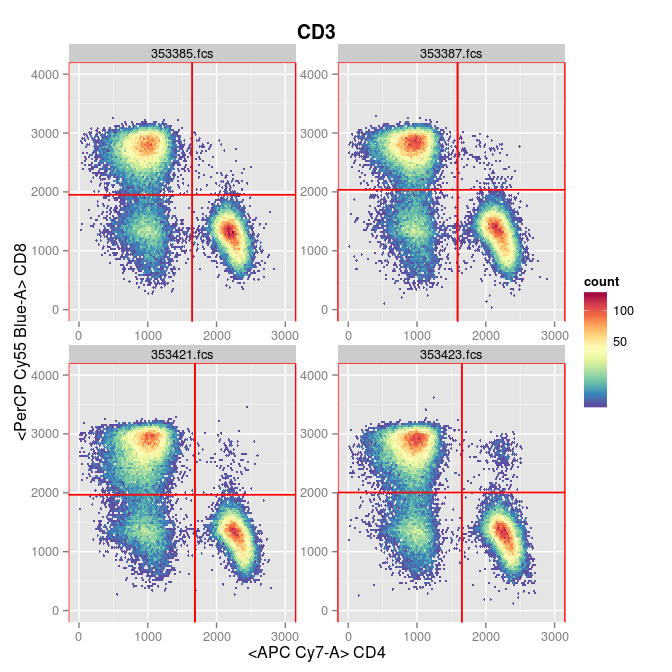### Next we will gate the cytokine-positive cells

In order to make inferences on the proportions of cytokine positive cells in the stimulated and non-stimulated samples within each subject, we should, ideally, apply the same gate to both samples within each subject. We can do this using the `groupBy` column in the template, to specify the Patient ID (`PID`) as the grouping variable, and set `collapseDataForGating` to `TRUE` in order to use both samples together to derive the gate.

The cytokines of interest are:

``kable(pData(parameters(getData(tbdata_subset[])))[,1:2],row.names=FALSE)``
name desc
Time NA
FSC-A NA
FSC-H NA
SSC-A NA
IFNg
AViD
CD14
IL2
CD3
CD154
PE Cy55-A NA
IL22
IL4
IL17a
CD4
TNFa
CD8

AViD, CD14, CD3, CD4 and CD8 are phenotypic markers. We want to gate IL2, IFNg, CD154, IL22, IL4, IL17a and TNFa marginally for CD4+/CD8- T cells.

#### TNFa

``````        template = rbind(
tbdata_subset,alias = "TNF",pop = "TNF+",dims = "TNFa",gating_method = "tailgate",gating_args = "auto_tol=TRUE",parent =
"CD4+CD8-",collapseDataForGating = TRUE,groupBy = "PID"
)
)
ggcyto(tbdata_subset,mapping = aes(x = "TNFa",y = "SSC-A"),subset = "CD4+CD8-") +
geom_hex(bins = 100) + ggcyto_par_set(limits = "instrument") + geom_gate() +
facet_grid(PID ~ Peptide,scale = "free") + geom_gate()``````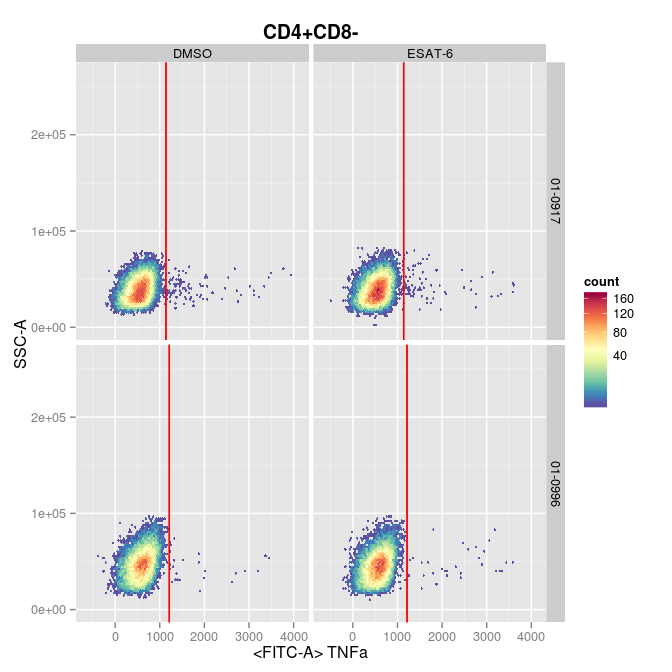#### IL2

``````          template = rbind(
tbdata_subset,alias = "IL2",pop = "IL2+",dims = "IL2",gating_method = "tailgate",gating_args = "auto_tol=TRUE",parent =
"CD4+CD8-",collapseDataForGating = TRUE,groupBy = "PID"
)
)
ggcyto(tbdata_subset,mapping = aes(x = "IL2",y = "SSC-A"),subset = "CD4+CD8-") +
geom_hex(bins = 100) + ggcyto_par_set(limits = "instrument") + geom_gate() +
facet_grid(PID ~ Peptide,scale = "free") + geom_gate()``````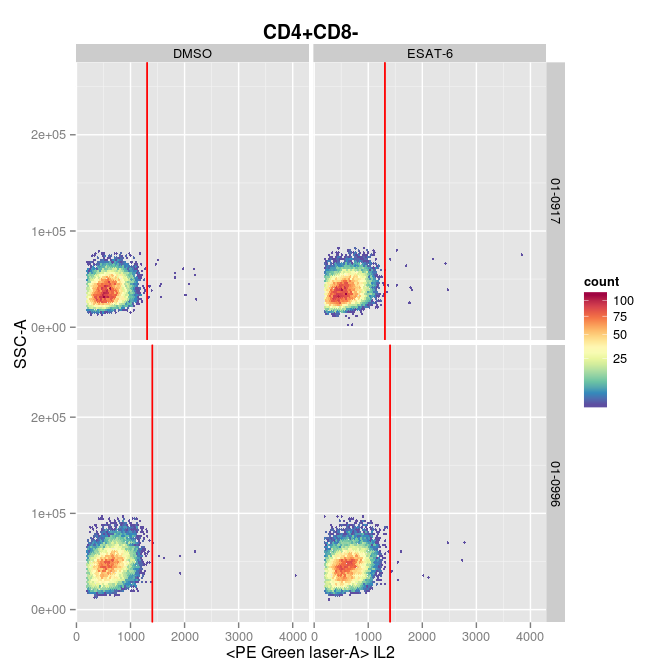#### IL22

``````          template = rbind(
tbdata_subset,alias = "IL22",pop = "IL22+",dims = "IL22",gating_method =
"tailgate",gating_args = "auto_tol=TRUE,adjust=2",parent = "CD4+CD8-",collapseDataForGating = TRUE,groupBy = "PID"
)
)
ggcyto(tbdata_subset,mapping = aes(x = "IL22",y = "SSC-A"),subset = "CD4+CD8-") +
geom_hex(bins = 100) + ggcyto_par_set(limits = "instrument") + geom_gate() +
facet_grid(PID ~ Peptide,scale = "free") + geom_gate()``````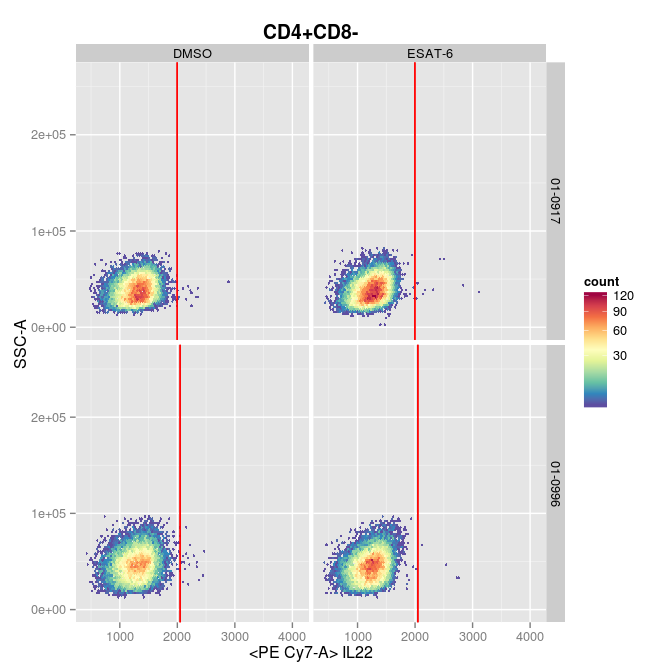#### IL4

``````          template = rbind(
tbdata_subset,alias = "IL4",pop = "IL4+",dims = "IL4",gating_method = "tailgate",gating_args = "auto_tol=TRUE",parent =
"CD4+CD8-",collapseDataForGating = TRUE,groupBy = "PID"
)
)
ggcyto(tbdata_subset,mapping = aes(x = "IL4",y = "SSC-A"),subset = "CD4+CD8-") +
geom_hex(bins = 100) + ggcyto_par_set(limits = "instrument") + geom_gate() +
facet_grid(PID ~ Peptide,scale = "free") + geom_gate()``````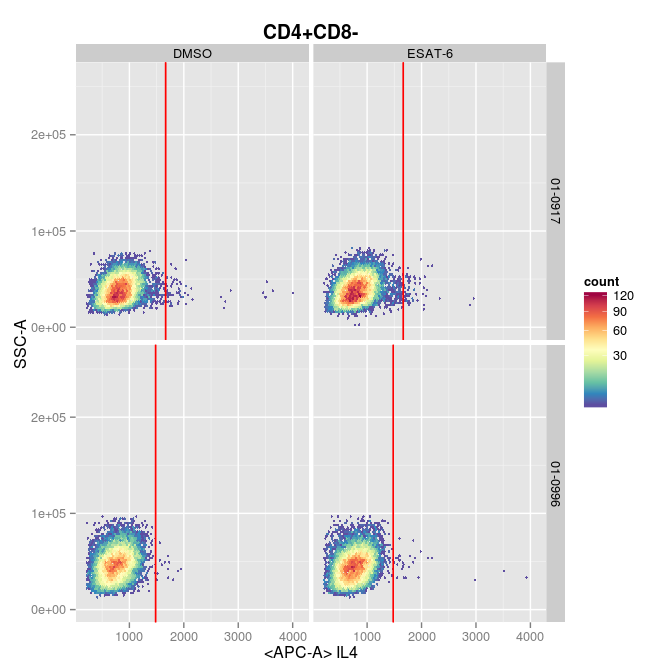#### CD154

``````            template = rbind(
tbdata_subset,alias = "CD154",pop = "CD154+",dims = "CD154",gating_method =
"tailgate",gating_args = "auto_tol=TRUE",parent = "CD4+CD8-",collapseDataForGating = TRUE,groupBy = "PID"
)
)
ggcyto(tbdata_subset,mapping = aes(x = "CD154",y = "SSC-A"),subset = "CD4+CD8-") +
geom_hex(bins = 100) + ggcyto_par_set(limits = "instrument") + geom_gate() +
facet_grid(PID ~ Peptide,scale = "free") + geom_gate()``````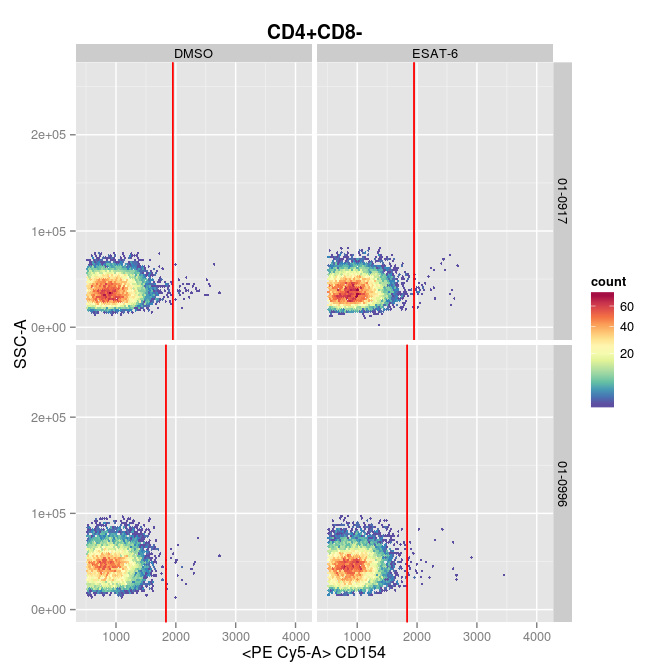#### IFNg

``````              template = rbind(